## Schedule

Keras and Neural Network Fundamentals

MLflow and Spark UDFs

Hyperparameter Tuning with Hyperopt

Horovod: Distributed Model Training

LIME, SHAP & Model Interpretability

CNNs and ImageNet

Transfer Learning

Object Detection

## Survey

Pandas/Spark?

Machine Learning? Deep Learning?

Expectations?

# Deep Learning Overview

## Why Deep Learning?

Performs well on complex datasets like images, sequences, and natural language

Scales better as data size increases

Theoretically can learn any shape (universal approximation theorem)

## Open Source Landscape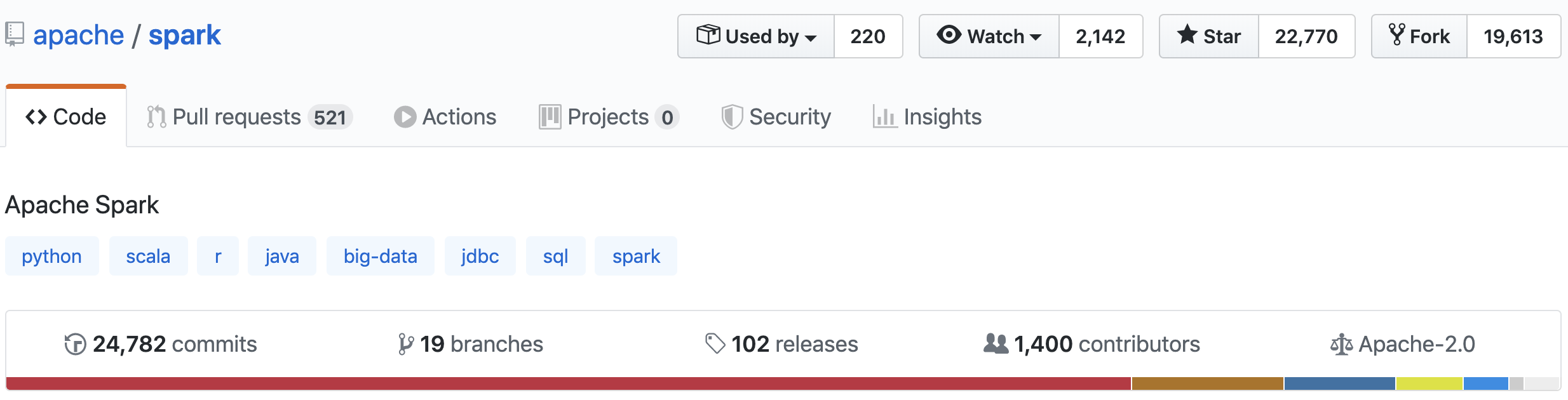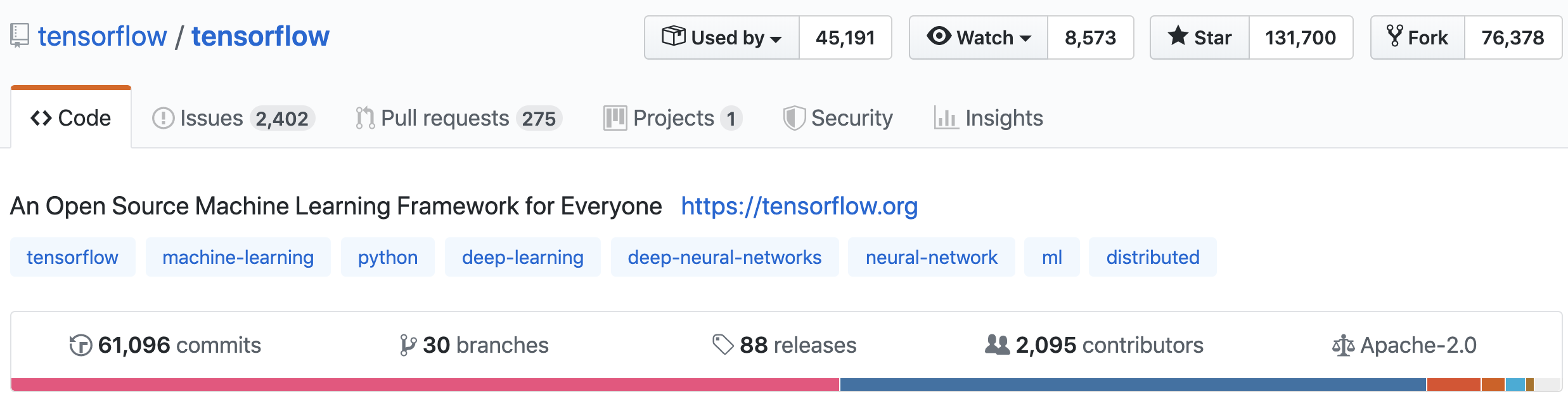## Where Does DL Fit In?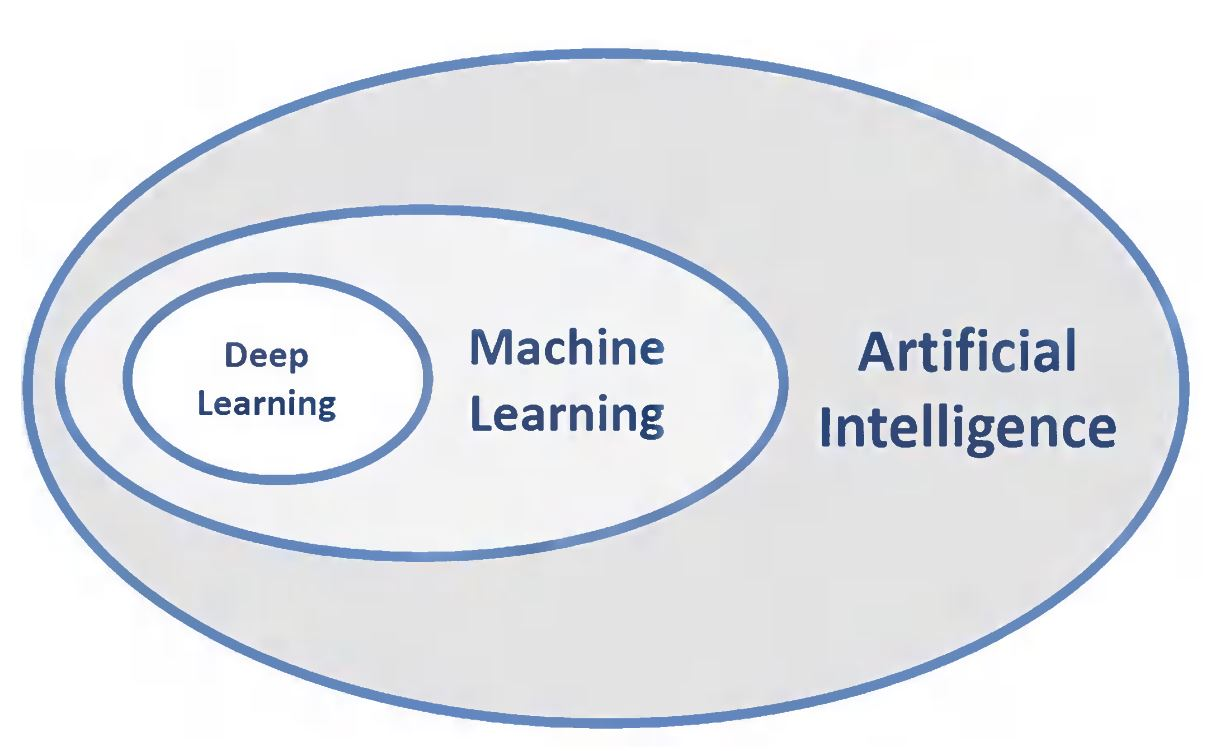## What is Deep Learning?

Composing representations of data in a hierarchical manner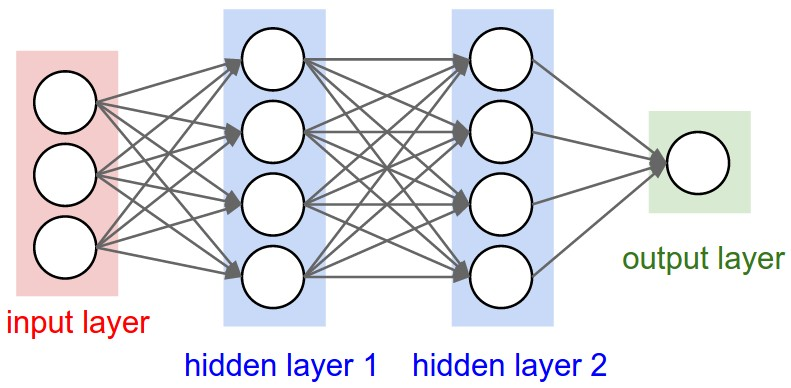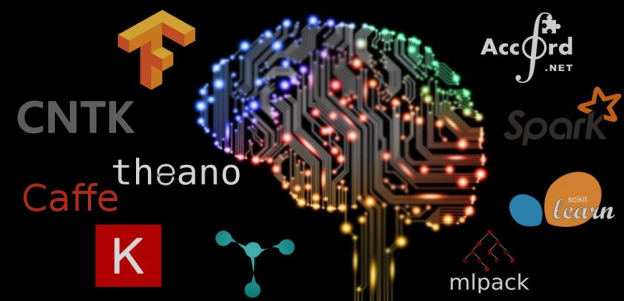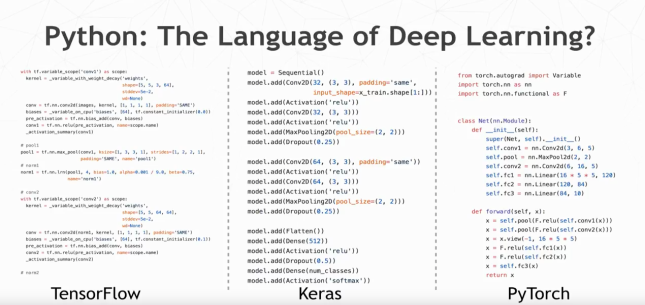## Keras

High-level Python API to build neural networks

Official high-level API of TensorFlow

Has over 250,000 users

Released by François Chollet in 2015

## Why Keras?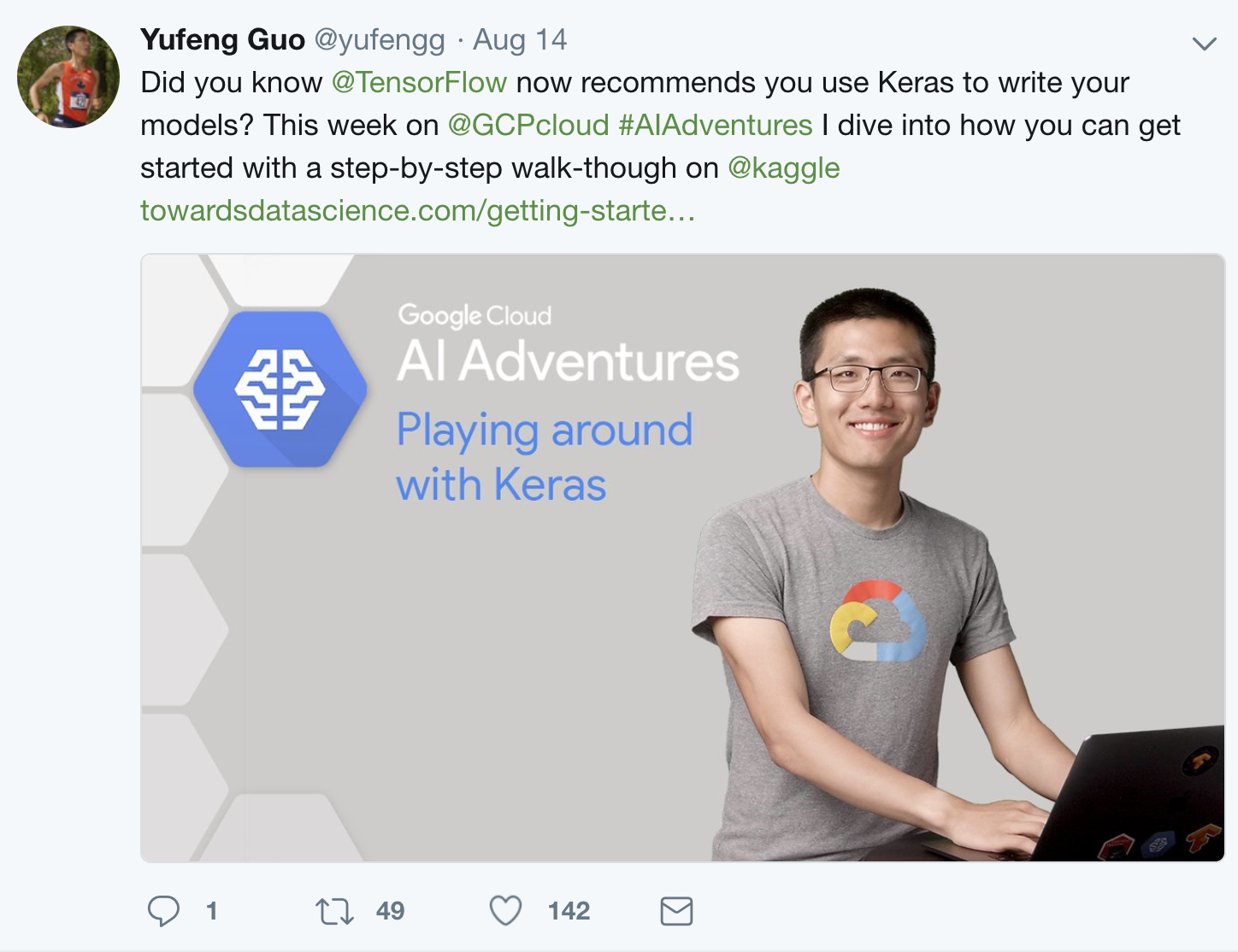## Hardware Considerations

GPUs are prefered for training due to speed of computation, but not good in data transfer

CPUs are generally acceptable for inference

## Why DL on Databricks?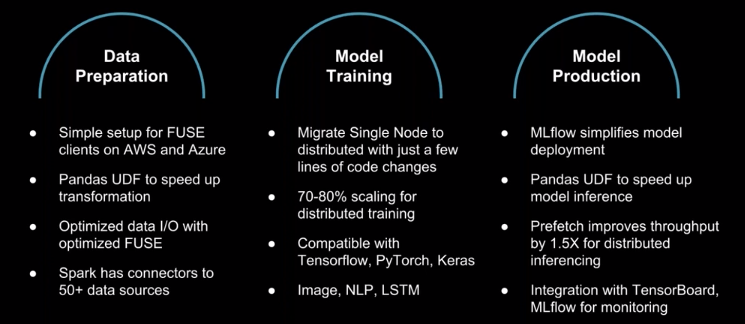## Layers

Input layer

Zero or more hidden layers

Output layer## Regression Evaluation

Measure "closeness" between label and prediction

• When predicting someone's weight, better to be off by 2 lbs instead of 20 lbs

Evaluation metrics:

• Loss: $(y - \hat{y})$
• Absolute loss: $|y - \hat{y}|$
• Squared loss: $(y - \hat{y})^2$

## Evaluation metric: MSE

$Error_{i} = (y_{i} - \hat{y_{i}})$

$SE_{i} = (y_{i} - \hat{y_{i}})^2$

$SSE = \sum_{i=1}^n (y_{i} - \hat{y_{i}})^2$

$MSE = \frac{1}{n}\sum_{i=1}^n (y_{i} - \hat{y_{i}})^2$

$RMSE = \sqrt{\frac{1}{n}\sum_{i=1}^n (y_{i} - \hat{y_{i}})^2}$

## Backpropagation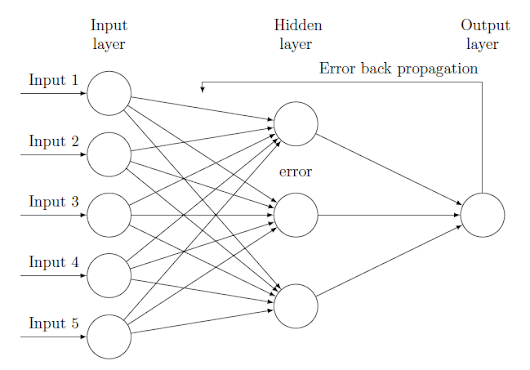Sigmoid

Tangent

ReLU

Leaky ReLU

PReLU

ELU

## Sigmoid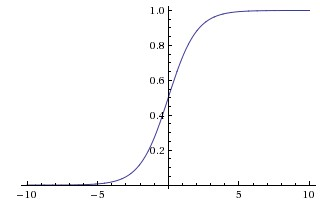Not zero-centered

## Hyperbolic tanget (Tanh)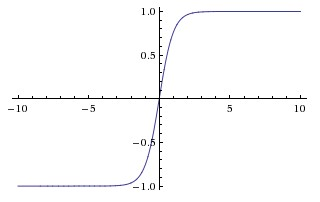Zero centered!

BUT, like the sigmoid, its activations saturate

## ReLU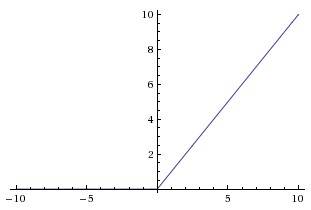BUT, gradients can still go to zero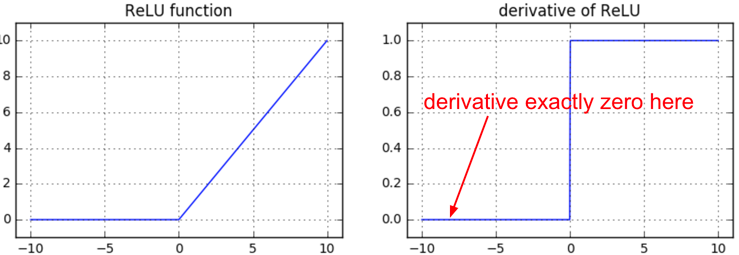## Leaky ReLU

For x < 0: $$f(x) = \alpha * x$$ For x >= 0: $$f(x) = x$$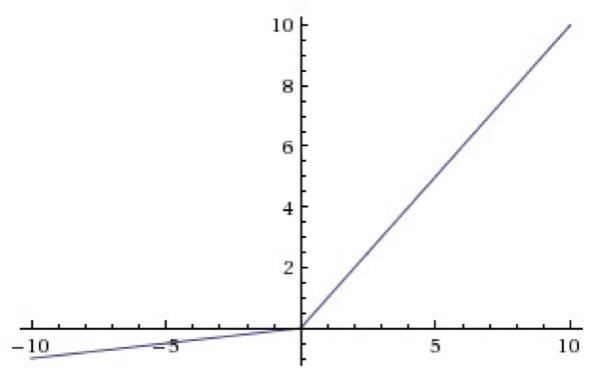These functions are not differentiable at 0, so we set the derivative to 0 or average of left and right derivative

## Comparison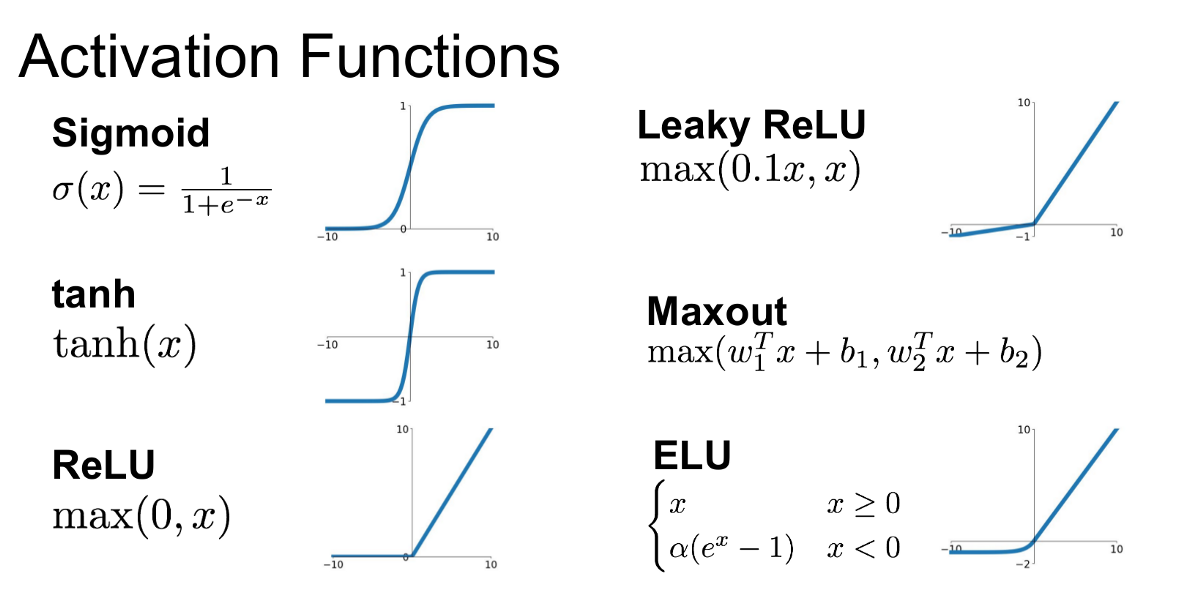## Optimizers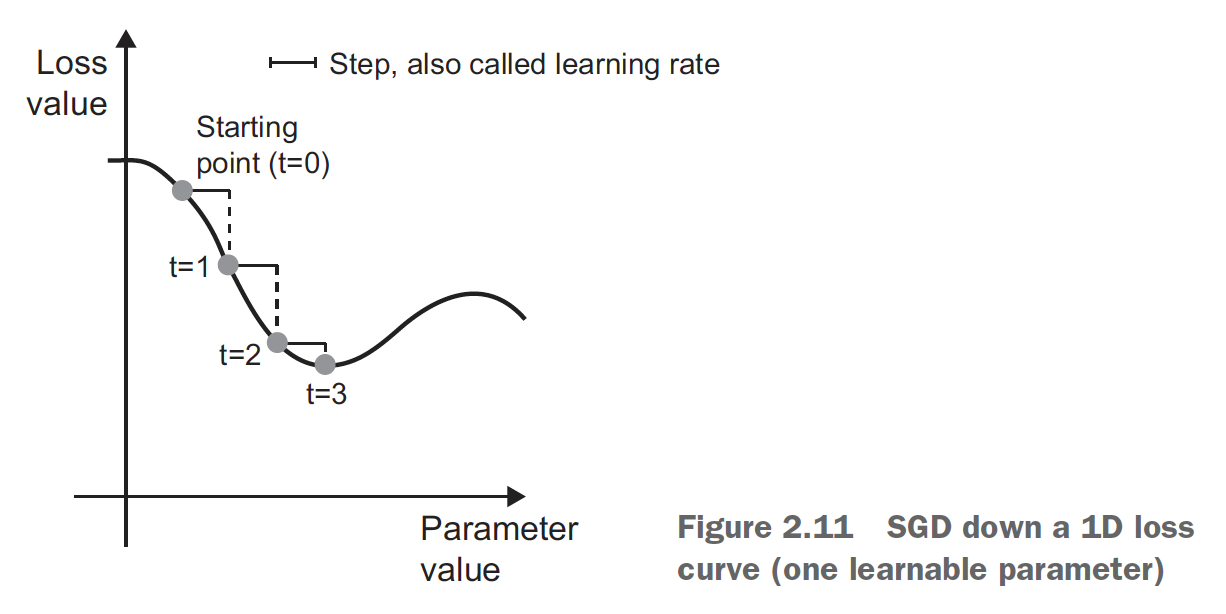Choosing a proper learning rate can be difficult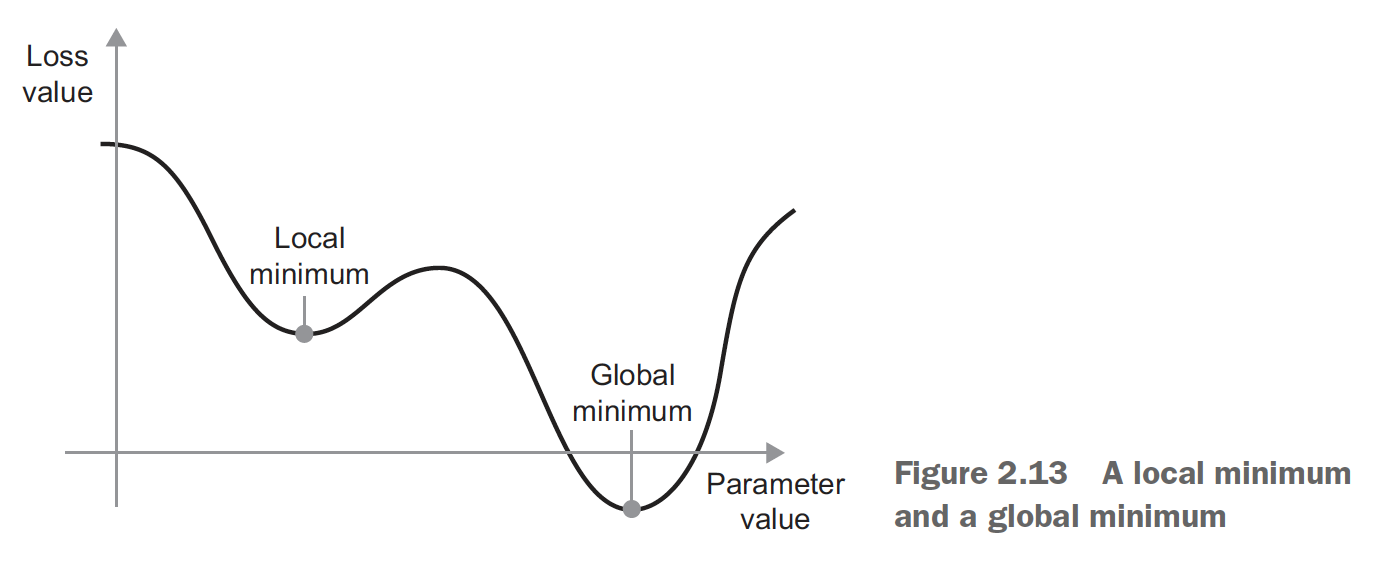Easy to get stuck in local minima

## Momentum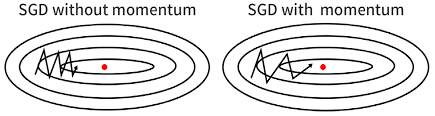Accelerates SGD: Like pushing a ball down a hill

Take average of direction we’ve been heading (current velocity and acceleration)

Limits oscillating back and forth, gets out of local minima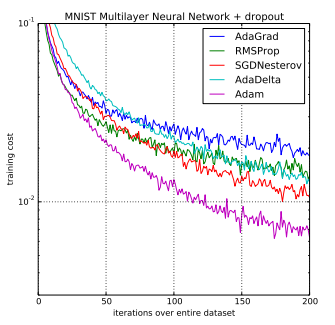## Hyperparameter Selection

Which dataset should we use to select hyperparameters? Train? Test?

## Validation Dataset

Split the dataset into three!

• Train on the training set
• Select hyperparameters based on performance of the validation set
• Test on test set

# Horovod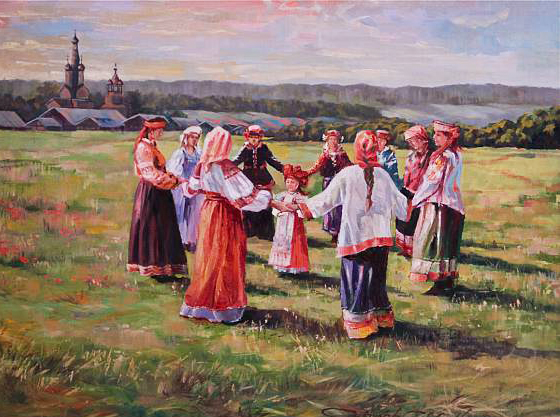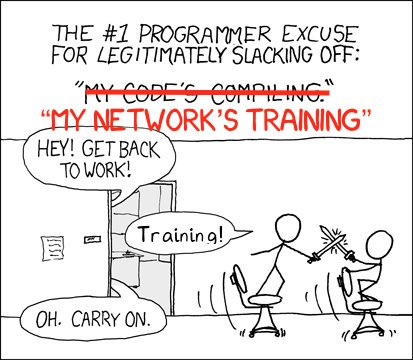## Horovod

Created by Alexander Sergeev of Uber, open-sourced in 2017

Simplifies distributed neural network training

Supports TensorFlow, Keras, PyTorch, and Apache MXNet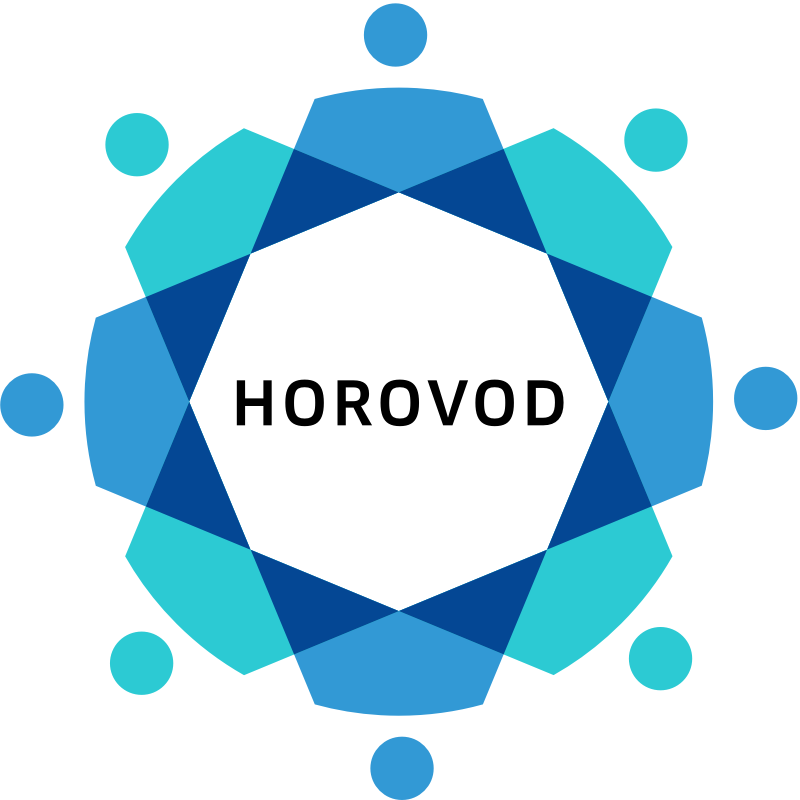## Classical Parameter Server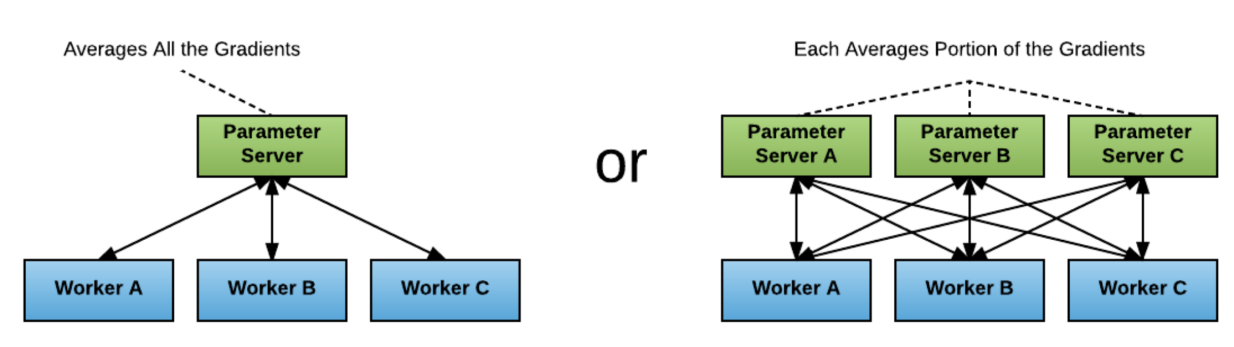## All-Reduce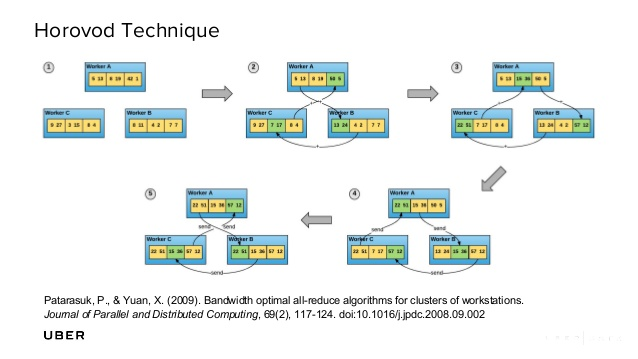# Only one line of code change!
optimizer = hvd.DistributedOptimizer(optimizer)


## LIME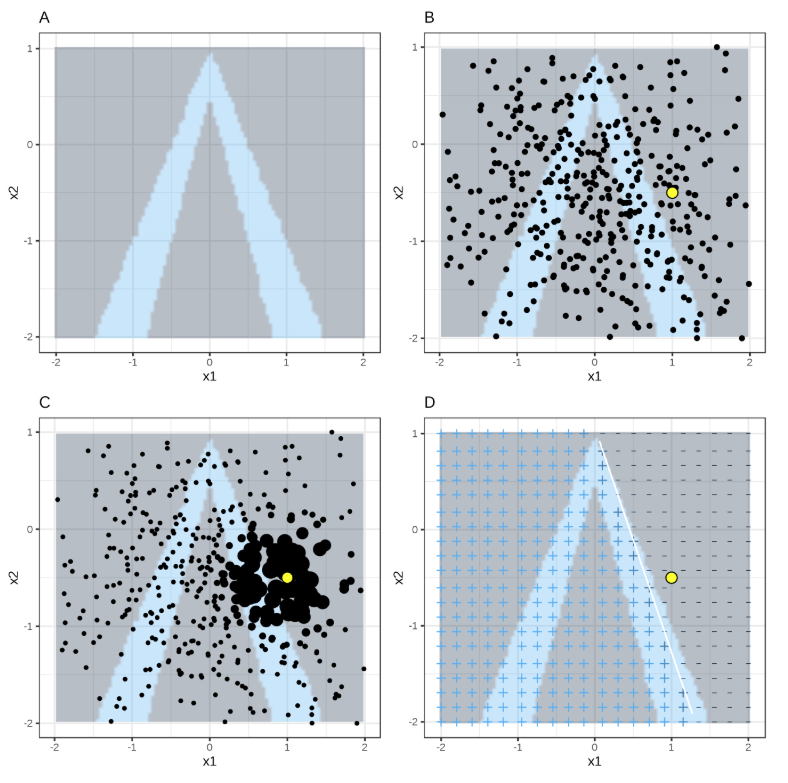## SHAP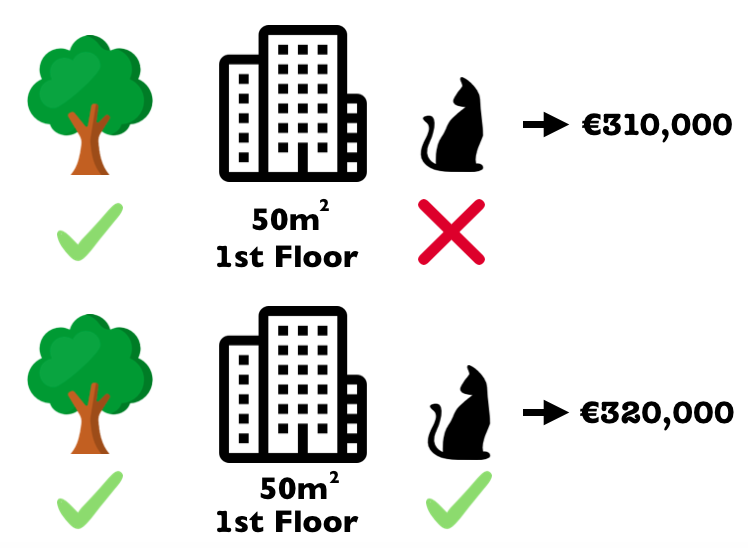Shapley Values

## SHAP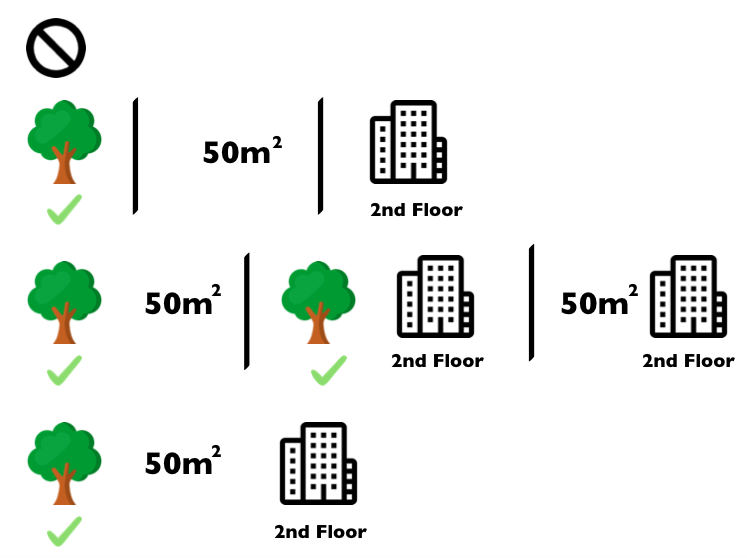## Convolutions

Focus on Local Connectivity (fewer parameters to learn)

Filter/kernel slides across input image (often 3x3)

Image Kernels Visualization

CS 231 Convolutional Networks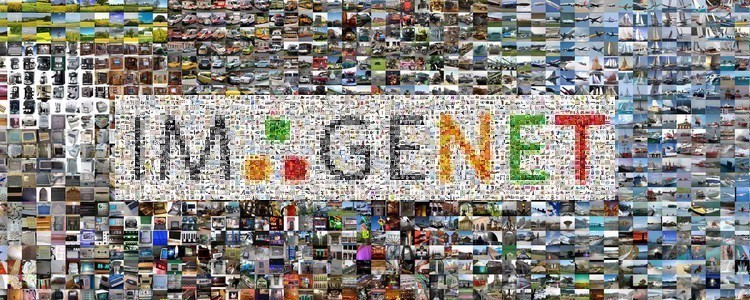## ImageNet Challenge

Classify images in one of 1000 categories

2012 Deep Learning breakthrough with AlexNet: 16% top-5 test error rate (next closest was 25%)

## VGG16 (2014)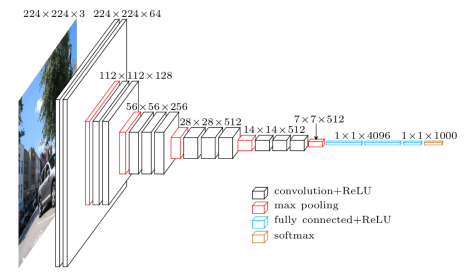One of the most widely used architectures for its simplicity

## Max vs Avg. Pooling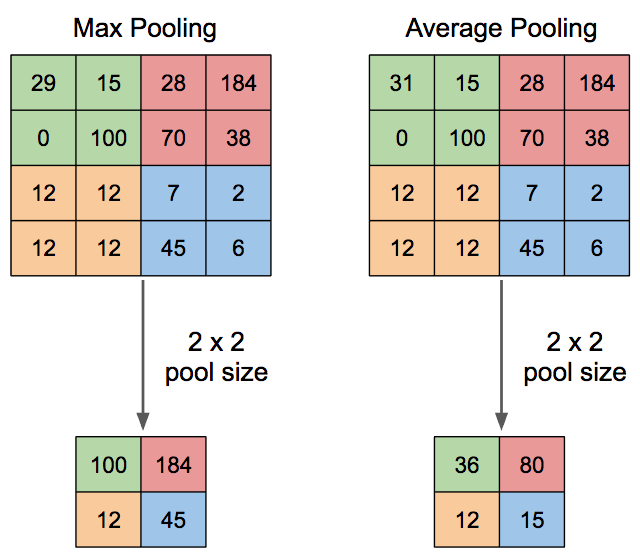## Inception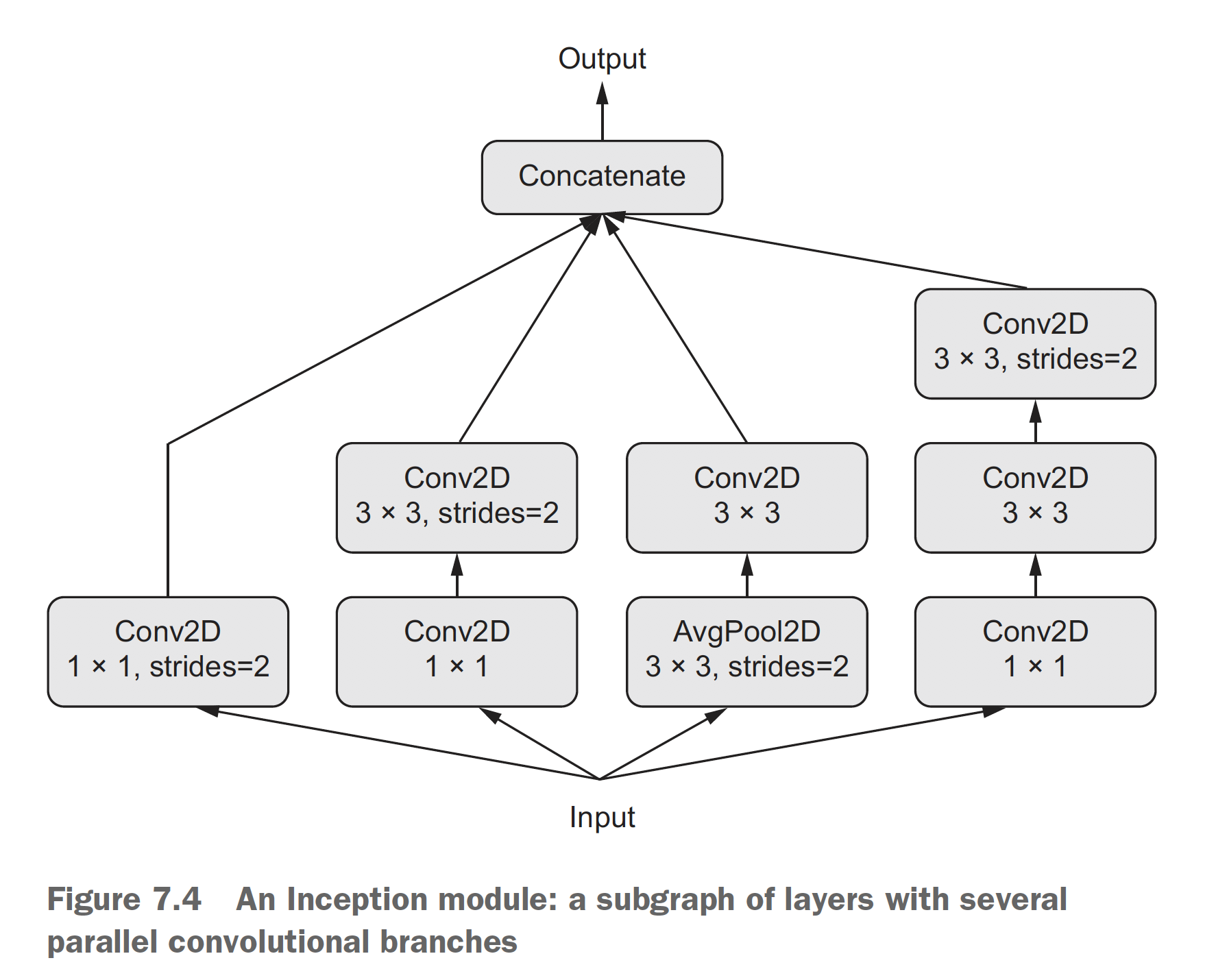## Residual Connection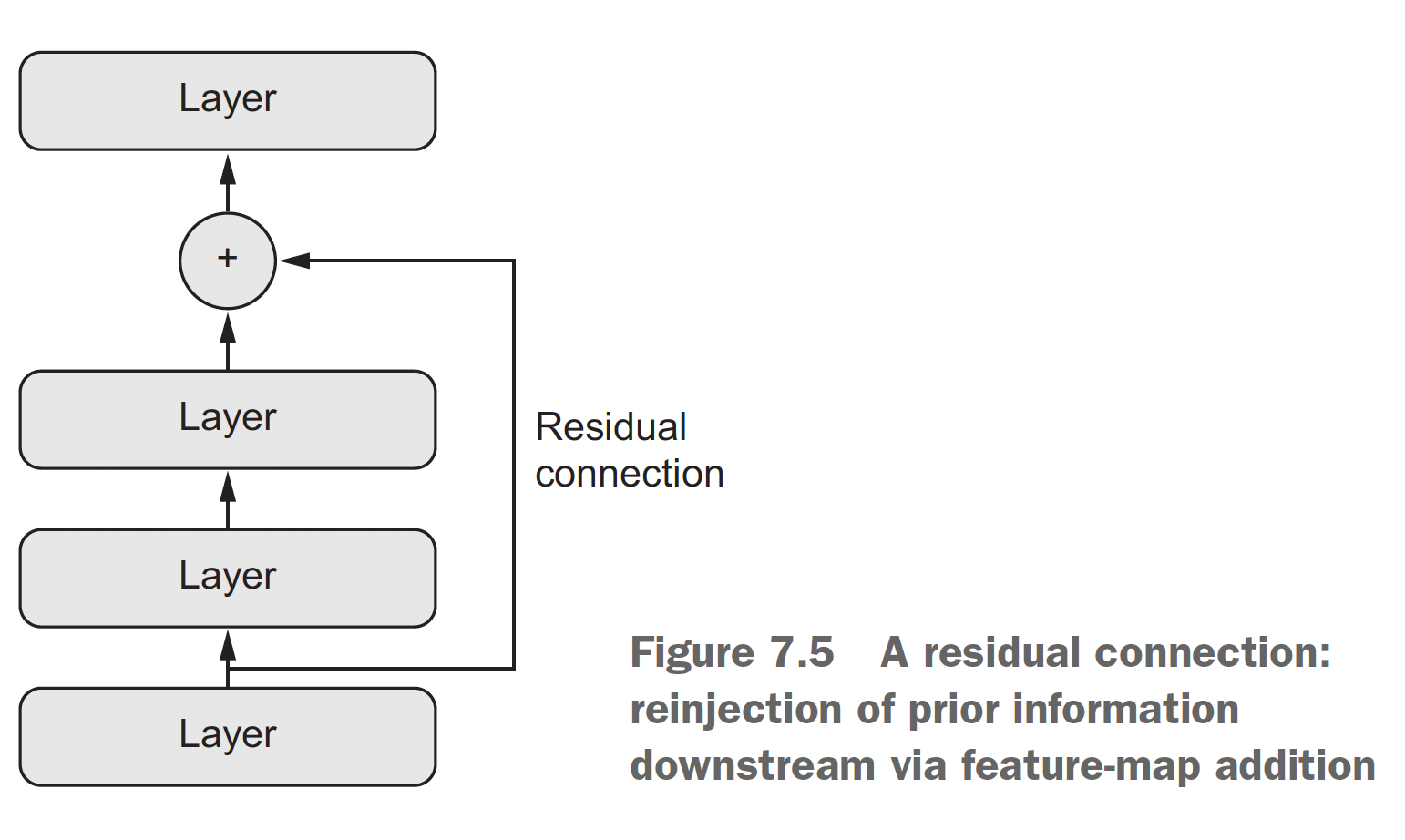## What do CNNs Learn?

Breaking Convnets

## Transfer Learning

IDEA: Intermediate representations learned for one task may be useful for other related tasks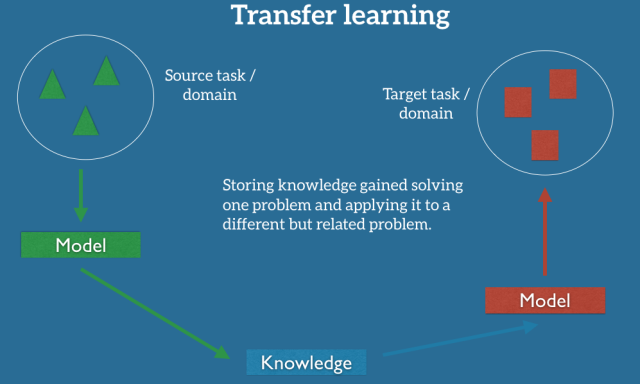### When to use Transfer Learning?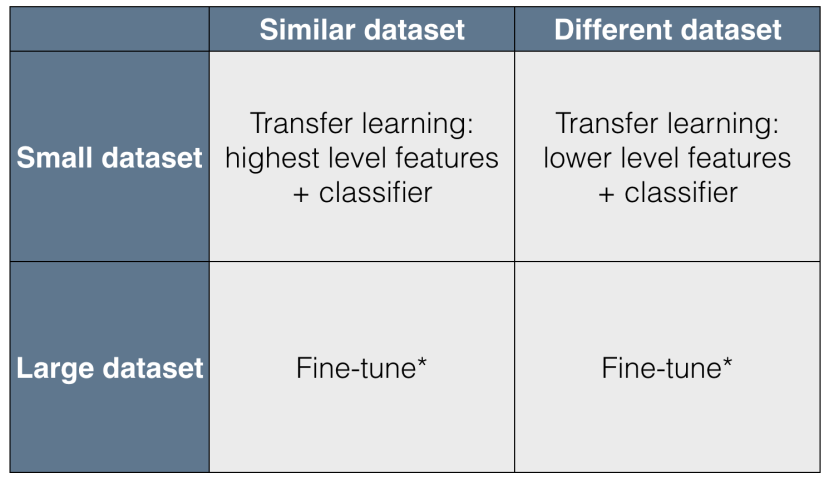## Transfer Learning

### Ok, so how do I find the optimal neural network architecture?

Neural Architecture Search with Reinforcement Learning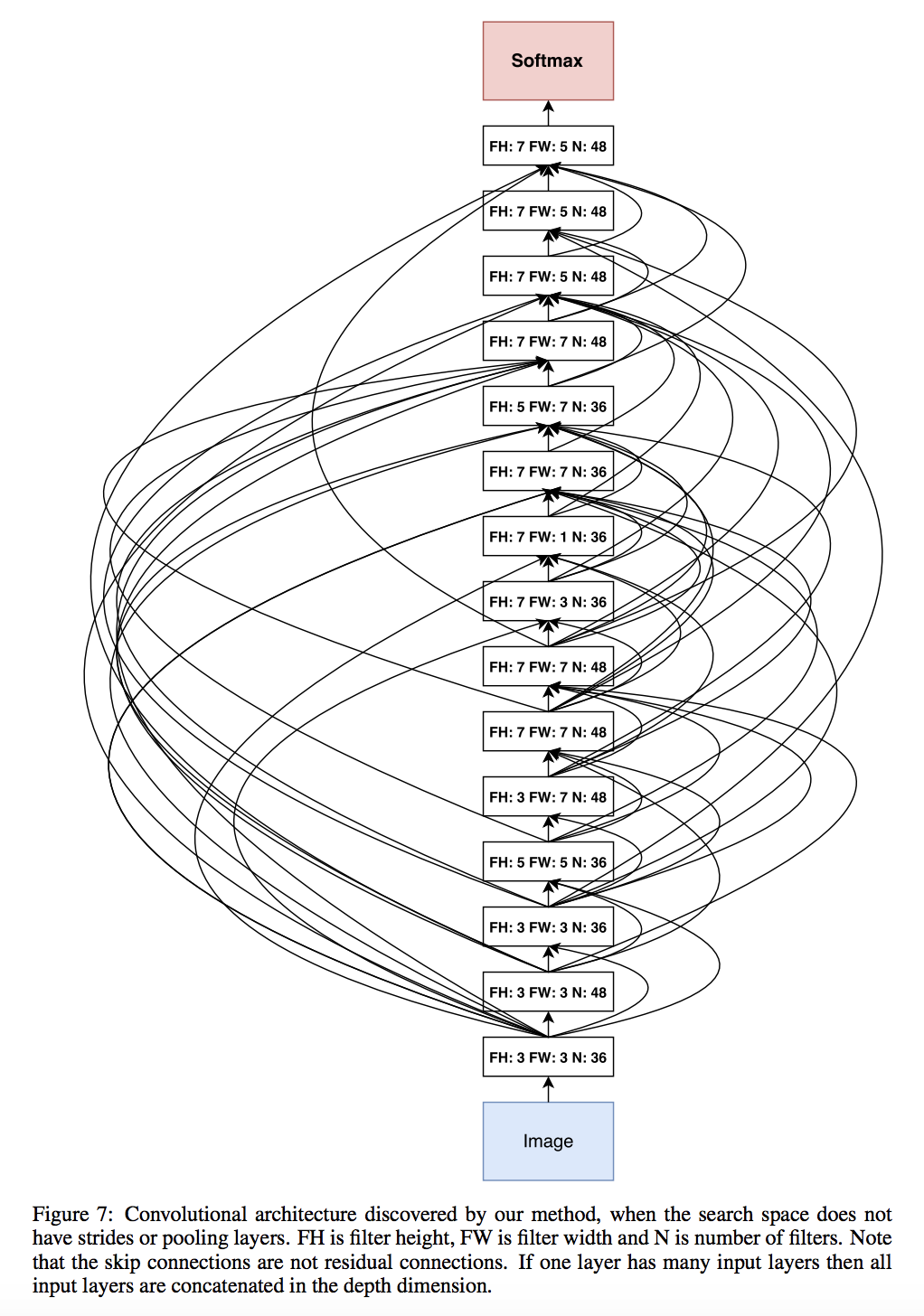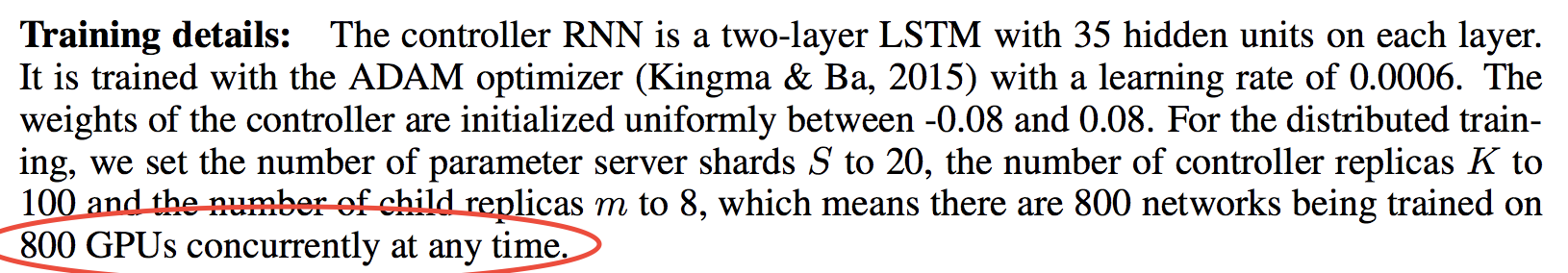Estimates generative models

Simultaneously trains two models

G: a generative model captures the data distribution

D: a discriminative model predicts probability of data coming from G

Used in generating art, deep fakes, up-scaling graphics, and astronomy research

## GANs Architecture: 2 Models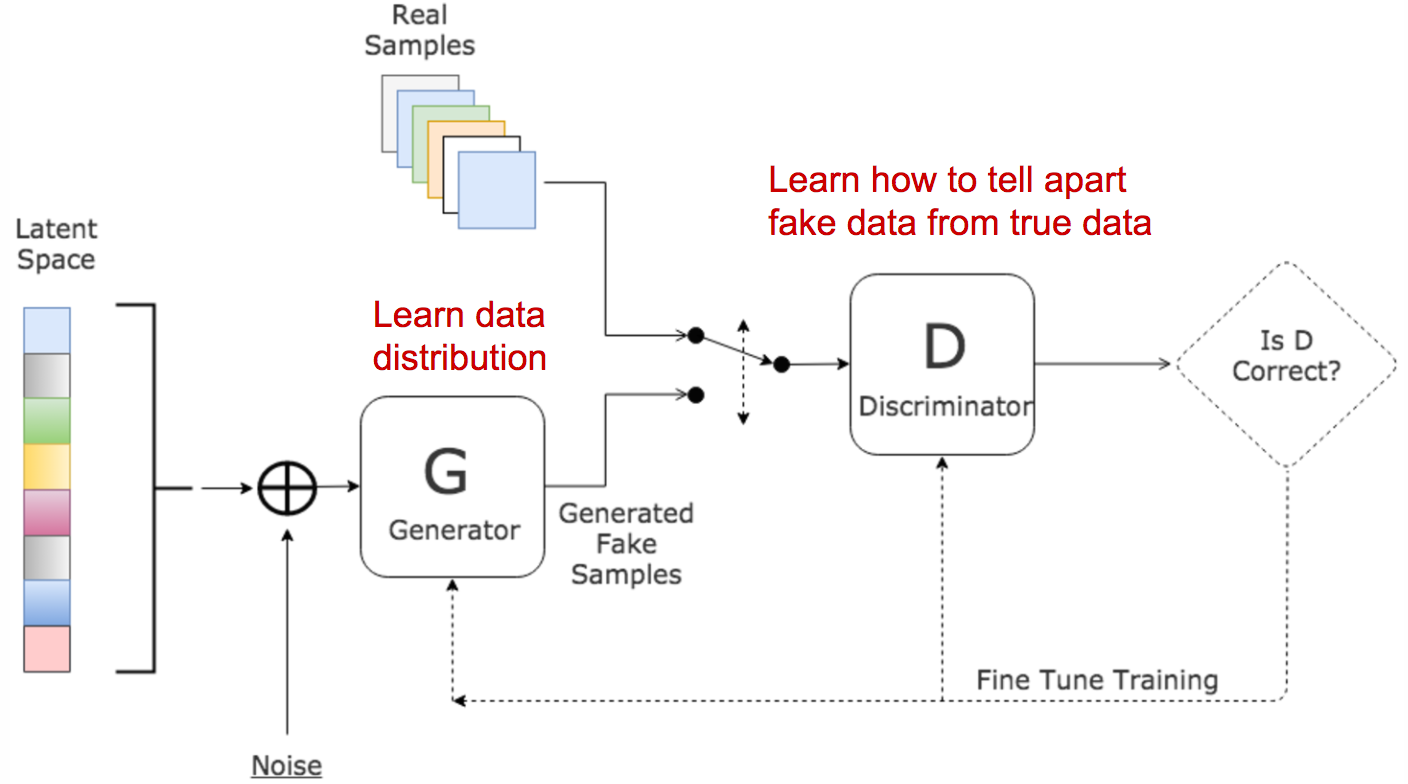## The Algorithm

G takes noise as input, outputs a counterfeit

D takes counterfeits and real values as input, outputs P(counterfeit)

To prevent overfitting...

• Alternate k steps of optimizing D and one step of optimizing G